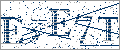>>

Login or Register   Integers - Simplification
 New User RegisterLogin Member: Email: Password:
 Site SearchAdvance Search

Other animationsIntegers on a Number Line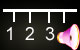Animation product List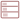Row format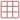Grid formatList formatOverview(For age - group : 11 - 16 )

Fully animated 'Integers - Simplification’ covers the concept using real life examples. This will help you to simplify complex expressions having vinculum, of and brackets. All the techniques are explained in a very detailed manner.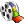Product - Animation
 Size/Duration Subscribe Preview / Trailer Size (KB) 2604 US\$    1.50 Rs.118.50  Check price in your currency Subscription Days = 30 Watch a preview (opens in separate window) Duration (hr:min:sec) 0:13:0 Add To Cart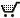This animation has audio.Category : Math Integer Type : Animation with sound Animation Type : Regular Total animation length: 13 minutes The animation covers: Addition of integers Substraction of integers Multiplication of integers Division of integers Simplication of integers Word problems in integers Quiz section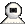Snapshots
 Watch combined previewWatch various movie clips related to integers. These are 1) Absolute value of an integer 2) Arithmetic operations of Integers 3) BODMAS Rule 4) Introduction to Integers 5) Number line - Representing History 6) Introduction to Natural and Whole Numbers 7) Representing Integers on Number line 8) Quiz on Integers. Click on the above thumbnails to go to the movie.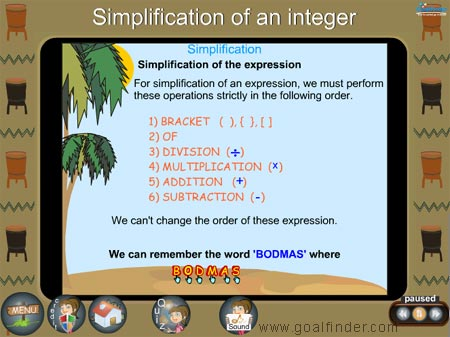The simplification of integers involves the application of BODMAS rule to the complex arithmetical expressions involving integers.This is like an animated math tutorial but with entertainment and stories to make learning integers a fun. We have provided many examples to clarify doubts in integers.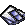Details of the animation/ movie /software

Solving Simplification of Integers with real life problems is the basis of the animation.

The simplification of integers section involves the use of BODMAS, a rule that enables us to know exactly the right sequence to perform an arithmetic operations.

(B)rackets (O)f (D)ivision (M)ultiplication (A)ddition (S)ubtraction

Example 1: What do you think the answer to 2 + 4 x 3 is?

6 x 3 = 18 or 2 + 12 = 14, here the BODMAS comes to the rescue, multiplication comes before addition so correct answer is
2 + 12 = 14

Example 2: 4 + 8 x 12 -25 = 4 + 96 -25 = 100 - 25 = 75

There is a quiz on word problems in integers with detailed solutions that will give a thorough understanding of the concept.

The animation covers the arithmetic operations of solving integers using real life examples; a quiz section with detailed solution also checks your understanding.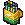Related Products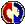Buyers Feedback:Give your comments